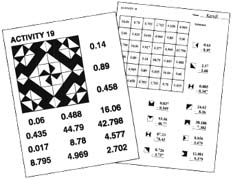# Jacobs Publishing "Design" Books

These math activity books contain worksheets that employ a search-and-shade technique in which students use a code to shade a grid on which answers to problems are found. If the exercise answers are correct, a symmetrical design develops. This allows the student to self check his or her work to a degree and provides a reward for working the problems. These popular books, used by thousands of math teachers across the USA, can also be used at home to review and reinforce learning.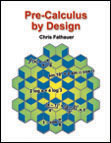Pre-Calculus by Design \$19.95 *** Temporarily Unavailable ***By Chris Fathauer. "Pre-Calculus by Design" contains 42 activity pages covering a range of trigonometry and other pre-calculus topics. These include trigonometric functions and laws, graphs of functions, working with logarithms and exponentials, arithmetic and geometric series, and limits. Click here to download the table of contents in pdf format.Click here to download a sample activity in pdf format.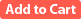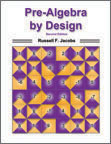Pre-Algebra by Design, 2nd Edition \$19.95 By Russell F. Jacobs. Contains 48 one-page activities to help bridge the gap between arithmetic and algebra. Click here to download the table of contents in pdf format.Click here to download a sample activity in pdf format.Algebra by Design \$19.95 By Russell F. Jacobs. Contains 44 one-page activities which provide a review of algebraic concepts and skills. The second edition, published in June of 2012, contains four new exercises on opposites and finding the missing term in a sequence. Click here to download the table of contents in pdf format.Click here to download a sample activity in pdf format.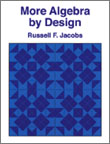More Algebra by Design, 2nd Edition \$19.95 By Russell F. Jacobs. Covers more advanced topics than "Algebra by Design", those often taught in the second semester of an algebra course. The second edition, new in 2011, includes four new exercises on the slope/intercept form of a line and linear inequalities in the coordinate plane. Click here to download the table of contents in pdf format.Click here to download a sample activity in pdf format.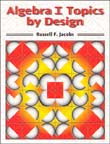Algebra I Topics by Design \$19.95 By Russell F. Jacobs. "Algebra I Topics by Design" contains 42 activity pages covering topics found in most first-year algebra programs. There is no repetition of exercises found in "Algebra by Design" and "More Algebra by Design", and in general the exercises in this new book are at a higher level of diffuculty. Click here to download the table of contents in pdf format.Click here to download a sample activity in pdf format.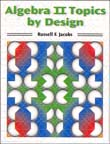Algebra II Topics by Design \$19.95 By Russell F. Jacobs. "Algebra II Topics by Design" contains 42 activity pages covering topics found in most second-year algebra courses. Concepts covered include exponential and logarithmic equations, summation notation, matrices and determinants, function notation, operations on functions, inverses of functions, and special functions such as absolute value and the greatest integer function. Click here to download the table of contents in pdf format.Click here to download a sample activity in pdf format.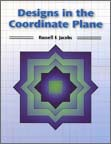Designs in the Coordinate Plane \$19.95 By Russell F. Jacobs. "Designs in the Coordinate Plane" brings a whole new twist to Jacobs Publishing's popular "Design" series. The student works math exercises which define ordered pairs of numbers that are then located as points in a cordinate plane. The student follows directions to connect the points so that a geometric design emerges. The mathematics exercises are at the level of first-year algebra and provide good review of order of operations, signed numbers, solving equtions, and more. Conatins 25 activities. Click here to download the table of contents in pdf format.Click here to download a sample activity in pdf format.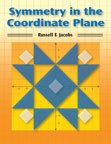Symmetry in the Coordinate Plane \$19.95 By Russell F. Jacobs. " Symmetry in the Coordinate Plane " contains 24 pairs of activity pages covering topics such as arithmetic operations on integers, order of operations, and solving equations. In each activity, students work a set of exercises that define a set of ordered pairs of numbers. These are located as labeled points in the coordinate plane. Directions are followed to connect the points, from which a design emerges, rewarding students for their efforts while allowing them to self-check their work. Teachers have permission to copy the pages for classroom use, and an answer key is provided. Grades 5-9. Click here to download the table of contents in pdf format.Click here to download a sample activity in pdf format.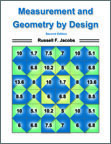Measurement and Geometry by Design, 2nd Edition \$19.95 By Russell F. Jacobs. Contains 44 activity pages ranging in content from the use of metric and customary units of measure to right triangle trigonometry. The Second Edition includes four new exercises on the geometry of solids. Click here to download the table of contents in pdf format.Click here to download a sample activity in pdf format.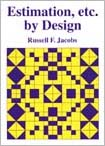Estimation, etc. by Design \$19.95 By Russell F. Jacobs. Covers rounding of numbers, estimating areas, identifying values of selected digits, measuring, and more. Click here to download the table of contents in pdf format.Click here to download a sample activity in pdf format.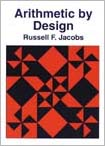Arithmetic by Design \$19.95 *** Not currently available *** By Russell F. Jacobs. Contains 48 one-page activities covering whole numbers, decimals, fractions, and percent. Click here to download the table of contents in pdf format.Click here to download a sample activity in pdf format.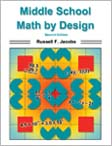Middle School Math by Design \$19.95 By Russell F. Jacobs. 40 pages of middle school math activities, including least common multiples, working with percents, interpreting linear graphs, and working with signed numbers. Click here to download the table of contents in pdf format.Click here to download a sample activity in pdf format.Grade 5 Math by Design \$19.95 *** Not available at this time *** By Russell F. Jacobs. 36 pages of Grade 5 math activities, including working with decimal numbers, simplifying fractions, and finding values from a straight line graph. Click here to download the table of contents in pdf format.Click here to download a sample activity in pdf format.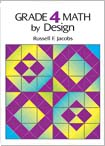Grade 4 Math by Design \$19.95 By Russell F. Jacobs. 36 pages of Grade 4 math activities, including addition, subtraction, multiplication, and division of 2- and 3-digit number, as well as measurement, word problems, rounding, and more. Click here to download the table of contents in pdf format.Click here to download a sample activity in pdf format.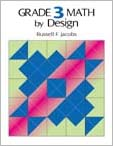Grade 3 Math by Design \$19.95 By Russell F. Jacobs. Follows up on "Grade 2 Math by Design" with exercises reinforcing Grade 3 math concepts. Click here to download the table of contents in pdf format.Click here to download a sample activity in pdf format.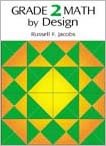Grade 2 Math by Design \$19.95 By Russell F. Jacobs. Provides review and practice of some of the crucial basic math skills for Grade 2, including addition, subtraction, multiplication, and division of whole numbers, counting by two's, five's, and ten's, and sums of money from pennies, nickels, dimes, and quarters. Click here to download the table of contents in pdf format.Click here to download a sample activity in pdf format.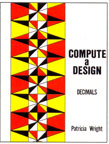Compute a Design  Decimals \$19.95 By Patricia Wright. Exercises cover addition, subtraction, multiplication, and division, with 15 exercises per page. Contains 48 activity pages and solution key.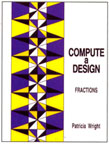Compute a Design  Fractions \$19.95 By Patricia Wright. Exercises cover basic concepts, reducing fractions, improper fractions, decimals, and mixed numbers, with 15 exercises per page. Contains 48 activity pages and solution key.Compute a Design  Percent \$19.95 By Patricia Wright. Exercises cover basic concepts, decimals as percents, percents as decimals, percents as fractions, and fractions as percent. Three cases are presented: percent of a number, unknown percent, and unknown number. Contains 48 pages of activities plus solution key, with 15 exercises per page.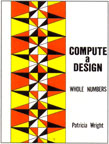Compute a Design  Whole Numbers \$19.95 By Patricia Wright. Exercises cover addition, subtraction, multiplication, and division, with 15 exercises per page. Contains 48 activity pages and solution key.Search n Shade \$19.95 By Pat Cornell. More than half the activities in this book are of the search-and-shade type featured in the other "Design" books, but it also contains four additional types of activities: Circlegrams, Coded Captions, Magic Squares, and Polyhedron Puzzles. Fifty-six activity pages cover addition, subtraction, multiplication, and division of whole numbers, decimals, fractions, and percent.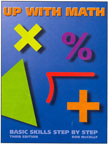Up With Math, Third Edition \$24.95 By Ron McCully. A step-by-step program to develop basic math skills, with chapters on whole numbers, perimeter and area, decimals, fractions, and percent. Up With Math teaches the detailed steps needed in computation. It focuses on an important subskill in each lesson. It explains each example clearly and patiently in a step-by-step style. It offers many practical applications as a realistic approach to problem solving. Student progress is evaluated with chapter self tests and a separate achievement testing program referenced to the performance objectives for each lesson. Paperback, 8-1/2" x 11", 286 pages.